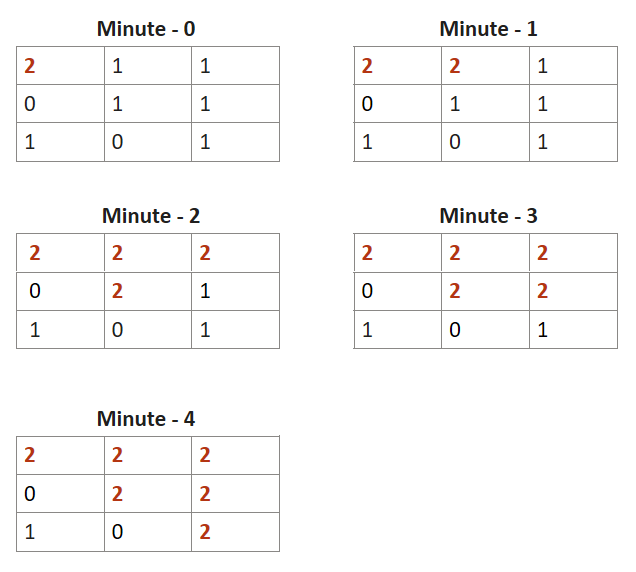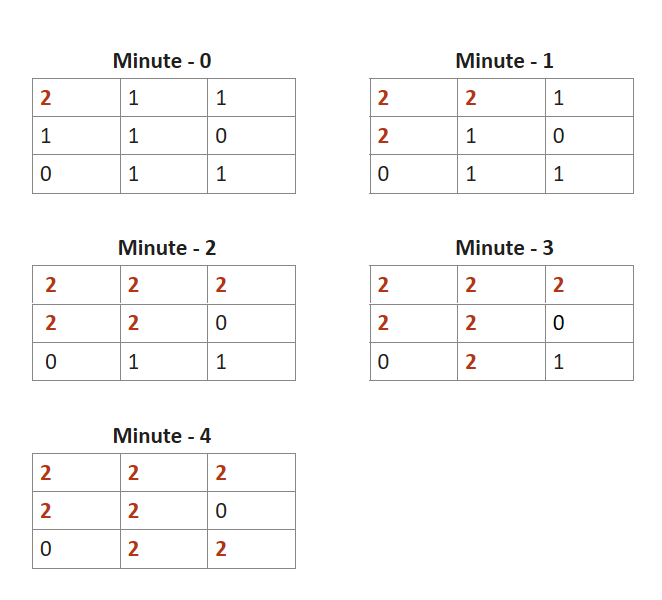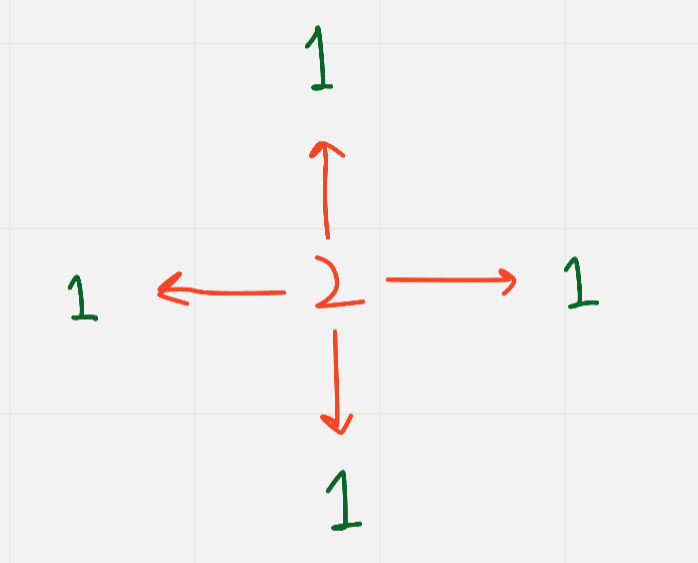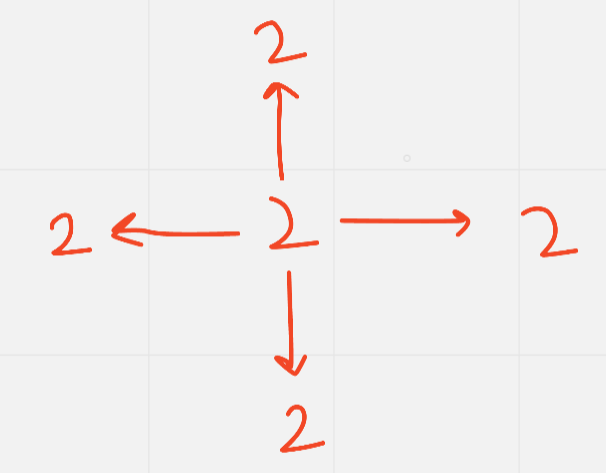# Rotten Oranges : Min time to rot all oranges : BFS

Problem Statement: You will be given an m x n grid, where each cell has the following values :

1. 2  –  represents a rotten orange
2. 1  –  represents a Fresh orange
3. 0  –  represents an Empty Cell

Every minute, if a Fresh Orange is adjacent to a Rotten Orange in 4-direction ( upward, downwards, right, and left ) it becomes Rotten.

Return the minimum number of minutes required such that none of the cells has a Fresh Orange. If it’s not possible, return -1.

Examples:

```Example 1:

Input: grid - [ [2,1,1] , [0,1,1] , [1,0,1] ]

Output: -1

Explanation:```
```Example 2:

Input: grid - [ [2,1,1] , [1,1,0] , [0,1,1] ]

Output:  4

Explanation:```

### Solution

Disclaimer: Don’t jump directly to the solution, try it out yourself first.

Intuition:

The idea is that for each rotten orange, we will find how many fresh oranges there are in its 4 directions. If we find any fresh orange we will make it into a rotten orange. One rotten orange can rotten up to 4 fresh oranges present in its 4 directions. For this problem,  we will be using the BFS ( Breadth-First Search ) technique.Approach:

-> First of all we will create a Queue data structure to store coordinate of Rotten Oranges

We will also have variables as:

1. Total_oranges – It will store total number of oranges in the grid ( Rotten + Fresh )
2. Count – It will store the total number of oranges rotten by us .
3. Total_time – total time taken to rotten.

-> After this, we will traverse the whole grid and count the total number of oranges in the grid and store it in Total_oranges. Then we will also push the rotten oranges in the Queue data structure as well.

-> Now while our queue is not empty,  we will pick up each Rotten Orange and check in all its 4 directions whether a Fresh orange is present or not. If it is present we will make it rotten and push it in our queue data structure and pop out the Rotten Orange which we took up as its work is done now.

-> Also we will keep track of the count of rotten oranges we are getting.

-> If we rotten some oranges, then obviously our queue will not be empty. In that case, we will increase our total time. This goes on until our queue becomes empty.

-> After it becomes empty, We will check whether the total number of oranges initially is equal to the current count of oranges. If yes, we will return the total time taken, else will return -1 because some fresh oranges are still left and can’t be made rotten.

Code:

## C++ Code

``````#include<bits/stdc++.h>
using namespace std;
int orangesRotting(vector<vector<int>>& grid) {
if(grid.empty()) return 0;
int m = grid.size(), n = grid.size(), days = 0, tot = 0, cnt = 0;
queue<pair<int, int>> rotten;
for(int i = 0; i < m; ++i){
for(int j = 0; j < n; ++j){
if(grid[i][j] != 0) tot++;
if(grid[i][j] == 2) rotten.push({i, j});
}
}

int dx = {0, 0, 1, -1};
int dy = {1, -1, 0, 0};

while(!rotten.empty()){
int k = rotten.size();
cnt += k;
while(k--){
int x = rotten.front().first, y = rotten.front().second;
rotten.pop();
for(int i = 0; i < 4; ++i){
int nx = x + dx[i], ny = y + dy[i];
if(nx < 0 || ny < 0 || nx >= m || ny >= n || grid[nx][ny] != 1) continue;
grid[nx][ny] = 2;
rotten.push({nx, ny});
}
}
if(!rotten.empty()) days++;
}

return tot == cnt ? days : -1;
}

int main()
{
vector<vector<int>> v{ {2,1,1} , {1,1,0} , {0,1,1} } ;
int rotting = orangesRotting(v);
cout<<"Minimum Number of Minutes Required "<<rotting<<endl;

}``````

Output:

Minimum Number of Minutes Required 4

Time Complexity: O ( n x n ) x 4

Reason: Worst-case – We will be making each fresh orange rotten in the grid and for each rotten orange will check in 4 directions

Space Complexity: O ( n x n )

Reason: worst-case –  If all oranges are Rotten, we will end up pushing all rotten oranges into the Queue data structure

## Java Code

``````import java.util.*;
class TUF{
public static int orangesRotting(int[][] grid) {
if(grid == null || grid.length == 0) return 0;
int rows = grid.length;
int cols = grid.length;
Queue<int[]> queue = new LinkedList<>();
int count_fresh = 0;
//Put the position of all rotten oranges in queue
//count the number of fresh oranges
for(int i = 0 ; i < rows ; i++) {
for(int j = 0 ; j < cols ; j++) {
if(grid[i][j] == 2) {
queue.offer(new int[]{i , j});
}
if(grid[i][j] != 0) {
count_fresh++;
}
}
}

if(count_fresh == 0) return 0;
int countMin = 0, cnt = 0;
int dx[] = {0, 0, 1, -1};
int dy[] = {1, -1, 0, 0};

//bfs starting from initially rotten oranges
while(!queue.isEmpty()) {
int size = queue.size();
cnt += size;
for(int i = 0 ; i < size ; i++) {
int[] point = queue.poll();
for(int j = 0;j<4;j++) {
int x = point + dx[j];
int y = point + dy[j];

if(x < 0 || y < 0 || x >= rows || y >= cols || grid[x][y] == 0 ||
grid[x][y] == 2) continue;

grid[x][y] = 2;
queue.offer(new int[]{x , y});
}
}
if(queue.size() != 0) {
countMin++;
}
}
return count_fresh == cnt ? countMin : -1;
}
public static void main(String args[])
{
int arr[][]={ {2,1,1} , {1,1,0} , {0,1,1} };
int rotting = orangesRotting(arr);
System.out.println("Minimum Number of Minutes Required "+rotting);
}
}``````

Output:

Minimum Number of Minutes Required 4

Time Complexity: O ( n x n ) x 4

Reason: Worst-case – We will be making each fresh orange rotten in the grid and for each rotten orange will check in 4 directions

Space Complexity: O ( n x n )

Reason: worst-case –  If all oranges are Rotten, we will end up pushing all rotten oranges into the Queue data structure

Special thanks to Shreyas Vishwakarma for contributing to this article on takeUforward. If you also wish to share your knowledge with the takeUforward fam, please check out this article